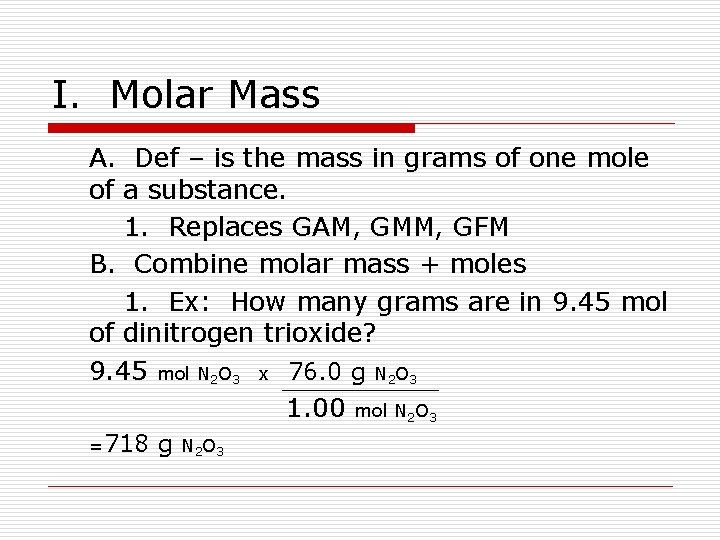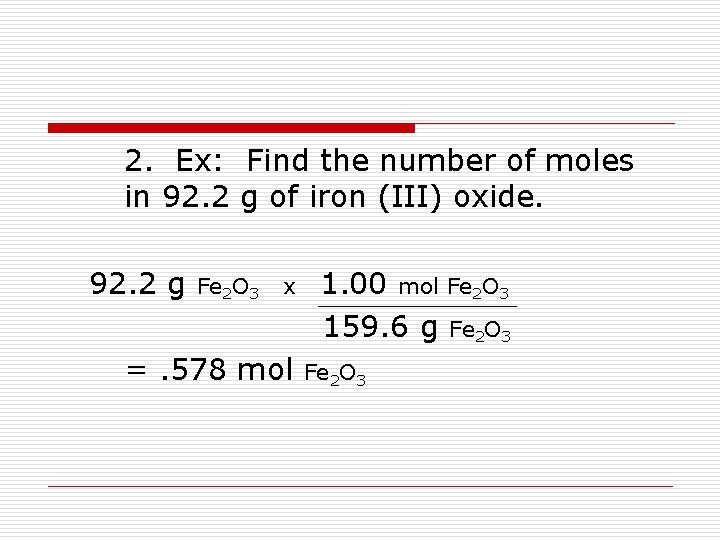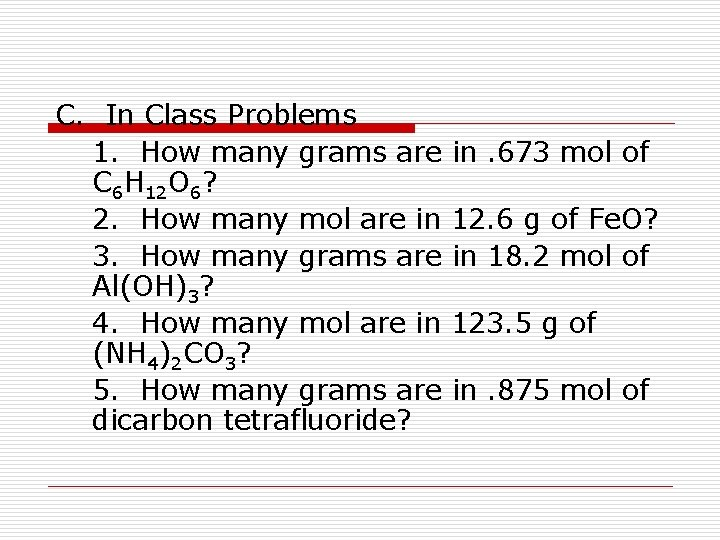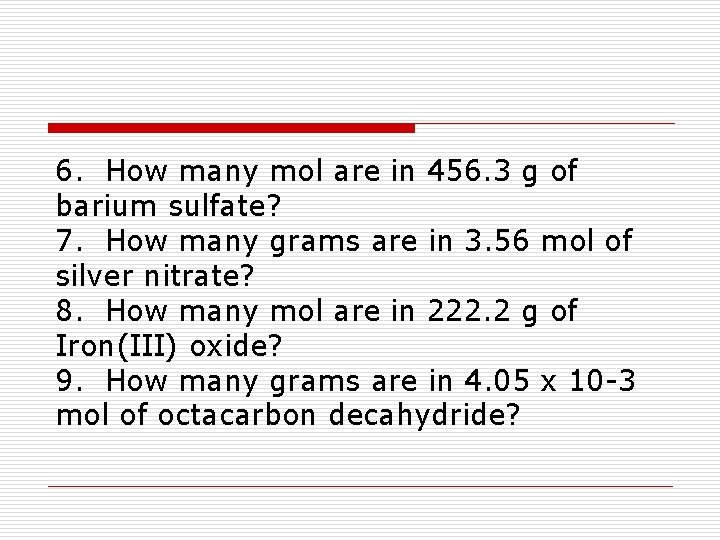# Chemistry 10 2 Mole Mass Mole Volume Relationships

• Slides: 8
Download presentationChemistry 10. 2 Mole – Mass / Mole – Volume RelationshipsI. Molar Mass A. Def – is the mass in grams of one mole of a substance. 1. Replaces GAM, GMM, GFM B. Combine molar mass + moles 1. Ex: How many grams are in 9. 45 mol of dinitrogen trioxide? 9. 45 mol N 2 O 3 x 76. 0 g N 2 O 3 1. 00 mol N 2 O 3 =718 g N 2 O 32. Ex: Find the number of moles in 92. 2 g of iron (III) oxide. 92. 2 g Fe 2 O 3 x =. 578 mol 1. 00 mol Fe 2 O 3 159. 6 g Fe 2 O 3C. In Class Problems 1. How many grams are C 6 H 12 O 6? 2. How many mol are in 3. How many grams are Al(OH)3? 4. How many mol are in (NH 4)2 CO 3? 5. How many grams are dicarbon tetrafluoride? in. 673 mol of 12. 6 g of Fe. O? in 18. 2 mol of 123. 5 g of in. 875 mol of6. How many mol are in 456. 3 g of barium sulfate? 7. How many grams are in 3. 56 mol of silver nitrate? 8. How many mol are in 222. 2 g of Iron(III) oxide? 9. How many grams are in 4. 05 x 10 -3 mol of octacarbon decahydride?II. The volume of a Mole of Gas A. Very Predictable 1. any gas… 1 mol = 22. 4 liters B. Gases vary with temperature and pressure. C. STP 1. Not “Stone Temple Pilots” 2. Standard Temperature and Pressure -0 deg. Celsius -1 AtmosphereD. Ex: Determine the volume of. 600 mol of SO 2 (g) at STP. . 600 mol of SO 2 =13. 4 L SO 2 x 22. 4 L SO 2 1 mol of SO 2E. The density of a gaseous compound containing carbon and oxygen is 1. 964 g/L at STP. Determine the molar mass of the compound. 1. 964 g 1 Liter x 22. 4 L 1 mol = 44. 0 g/mol